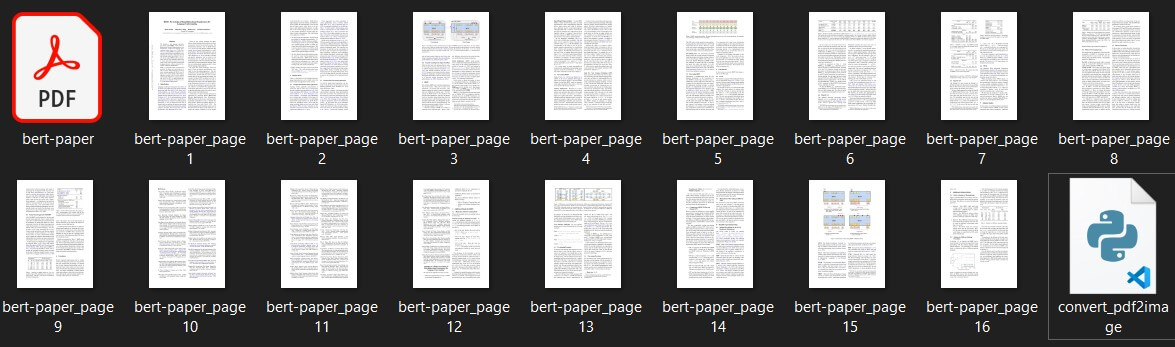# How to Convert PDF to Images in Python

Learn how to use PyMuPDF library to convert PDF files into individual images per page in Python.
4 min read · Updated jul 2022 · PDF File Handling

Disclosure: This post may contain affiliate links, meaning when you click the links and make a purchase, we receive a commission.

There are various tools to convert PDF files into images, such as pdftoppm in Linux. This tutorial aims to develop a lightweight command-line tool in Python to convert PDF files into images.

We'll be using PyMuPDF, a highly versatile, customizable PDF, XPS, and eBook interpreter solution that can be used across a wide range of applications such as a PDF renderer, viewer, or toolkit.

First, let's install the required library:

``\$ pip install PyMuPDF==1.18.9``

Importing the libraries:

``````import fitz

from typing import Tuple
import os``````

Let's define our main utility function:

``````def convert_pdf2img(input_file: str, pages: Tuple = None):
"""Converts pdf to image and generates a file by page"""
# Open the document
pdfIn = fitz.open(input_file)
output_files = []
# Iterate throughout the pages
for pg in range(pdfIn.pageCount):
if str(pages) != str(None):
if str(pg) not in str(pages):
continue
# Select a page
page = pdfIn[pg]
rotate = int(0)
# PDF Page is converted into a whole picture 1056*816 and then for each picture a screenshot is taken.
# zoom = 1.33333333 -----> Image size = 1056*816
# zoom = 2 ---> 2 * Default Resolution (text is clear, image text is hard to read)    = filesize small / Image size = 1584*1224
# zoom = 4 ---> 4 * Default Resolution (text is clear, image text is barely readable) = filesize large
# zoom = 8 ---> 8 * Default Resolution (text is clear, image text is readable) = filesize large
zoom_x = 2
zoom_y = 2
# The zoom factor is equal to 2 in order to make text clear
# Pre-rotate is to rotate if needed.
mat = fitz.Matrix(zoom_x, zoom_y).preRotate(rotate)
pix = page.getPixmap(matrix=mat, alpha=False)
output_file = f"{os.path.splitext(os.path.basename(input_file))}_page{pg+1}.png"
pix.writePNG(output_file)
output_files.append(output_file)
pdfIn.close()
summary = {
"File": input_file, "Pages": str(pages), "Output File(s)": str(output_files)
}
# Printing Summary
print("## Summary ########################################################")
print("\n".join("{}:{}".format(i, j) for i, j in summary.items()))
print("###################################################################")
return output_files``````

The above function converts a PDF file into a series of image files. It iterates through the selected pages (default is all of them), takes a screenshot of the current page, and generates an image file using the `writePNG()` method.

You can change the `zoom_x` and `zoom_y` to change the zoom factor, feel free to tweak these parameters and `rotate` variable to suit your needs.

Let's use this function now:

``````if __name__ == "__main__":
import sys
input_file = sys.argv
convert_pdf2img(input_file)``````

Let's test the script out on a multiple-page PDF file (get it here):

``\$ python convert_pdf2image.py bert-paper.pdf``

The output will be as the following:

``````## Summary ########################################################
File:bert-paper.pdf
Pages:None
Output File(s):['bert-paper_page1.png', 'bert-paper_page2.png', 'bert-paper_page3.png', 'bert-paper_page4.png', 'bert-paper_page5.png', 'bert-paper_page6.png', 'bert-paper_page7.png', 'bert-paper_page8.png', 'bert-paper_page9.png', 'bert-paper_page10.png', 'bert-paper_page11.png', 'bert-paper_page12.png', 'bert-paper_page13.png', 'bert-paper_page14.png', 'bert-paper_page15.png', 'bert-paper_page16.png']
###################################################################``````

And indeed, the images were successfully generated:

##Conclusion

We hope that you find this tutorial helpful for your needs, here are some other PDF tutorials:

Finally, if you're a beginner and want to learn Python, I suggest you take the Python For Everybody Coursera course, in which you'll learn a lot about Python. You can also check our resources and courses page to see the Python resources I recommend on various topics!

Check the full code here.

Happy coding ♥

Sharing is caring!

### Comment panel